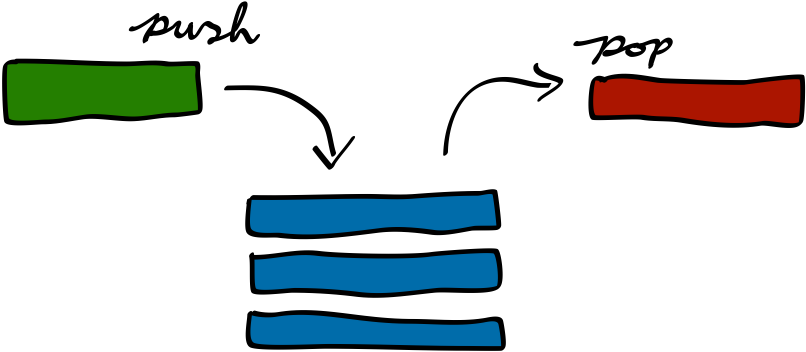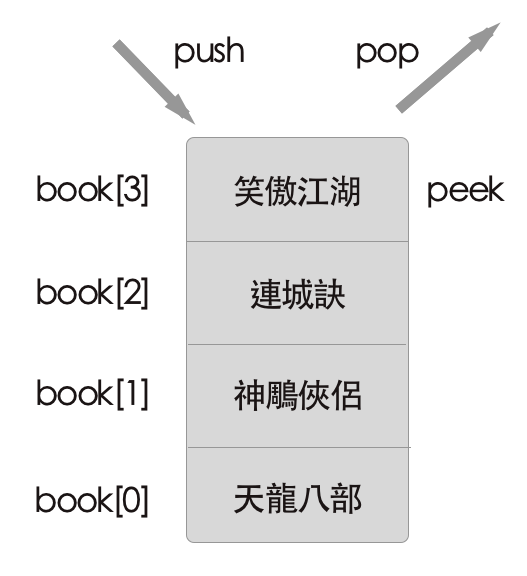# 用 JavaScript 學習資料結構和演算法：堆疊（Stack）篇# 建立堆疊

function Stack() {
// 類別內部宣告 Stack 的屬性和方法
}


1. let items = []; // 我們這邊使用陣列 array 的方式來儲存堆疊內的元素

1. push(elements)：新增一個或多個元素到堆疊頂部
2. pop()：移除堆疊頂部元素，同時返回被移除的元素
3. peek()top()：僅返回堆疊元素，不做任何修改
4. isEmpty()：檢查堆疊是否為空？若堆疊內無任何元素即返回 true，反之返回 false
5. clear()：清空堆疊裡的所有元素
6. size()：返回堆疊裡的元素個數，類似於陣列的 length 屬性

## 1. push(elements)、pop()

function Stack() {
let items = [];
this.push = function(element) {
items.push(element);
}
this.pop = function() {
return items.pop();
}
}## 2. peek()

function Stack() {
let items = [];
this.peek = function() {
return items[items.length - 1];
}
}


## 3. isEmpty()

function Stack() {
let items = [];
this.isEmpty = function() {
return items.length === 0;
}
}


## 4. clear()

function Stack() {
let items = [];
this.clear = function() {
items = [];
}
}


## 5. size()

function Stack() {
let items = [];
this.size = function() {
return items.length;
}
}


## 完整程式碼

function Stack() {
var items = [];
this.push = function(element) {
items.push(element);
}
this.pop = function() {
return items.pop();
}
this.peek = function() {
return items[items.length - 1];
}
this.isEmpty = function() {
return items.length === 0;
}
this.clear = function() {
items = [];
}
this.size = function() {
return items.length;
}
// 加入印出結果方法
this.print = function() {
console.log(items.toString());
}
}


# 成果展示

// 先貼上上述完整 Stack 類別，然後 new 一個物件
var stack = new Stack();
stack.push('松下問童子');
stack.push('言師採藥去');
stack.push('只在此山中');
stack.push('雲深不知處');
stack.pop();

// 接下來我們將可以透過 stack 物件來操作堆疊的方法：

console.log(stack.size()); // 你答對了嗎？
console.log(stack.peek());
stack.clear();
console.log(stack.isEmpty());


# 總結

stack.push('葡萄美酒夜光杯');
stack.push('欲飲琵琶馬上催');
stack.push('古來征戰幾人回');
stack.pop();
stack.push('醉臥沙場君莫笑');

# 延伸閱讀

(image via stack-machine)

@kdchang 文藝型開發者，夢想是做出人們想用的產品和辦一所心目中理想的學校。A Starter & Maker. JavaScript, Python & Arduino/Android lover.:)